# Potential Inside and Outside of a Charged Spherical Shell

• jkthejetplane

#### jkthejetplane

Homework Statement
I need help on this question that i thought i understood but i really dont. A semi similar example in the book started to spark some brain juice but i ended up more confused than when i started.
Relevant Equations
Legendre polynomials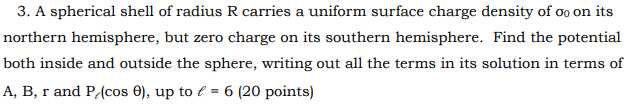So here was my first go around at it: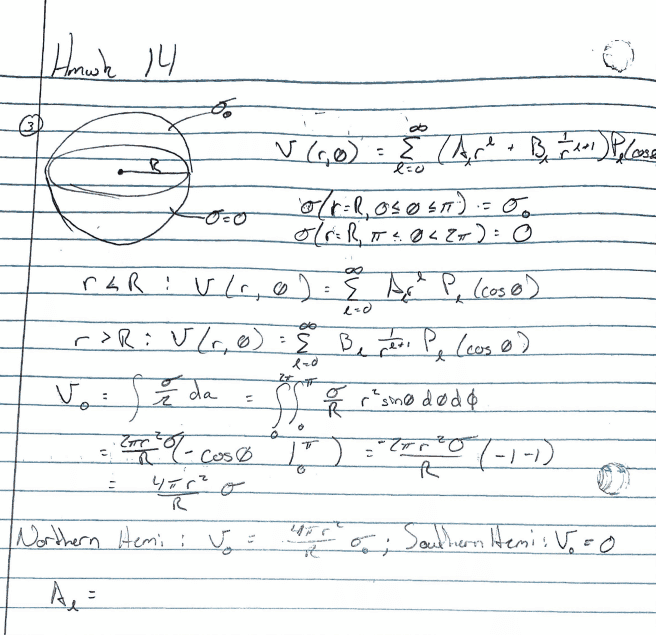At first it made sense in my head but don't think my process is correct. Then i noticed the example in the book: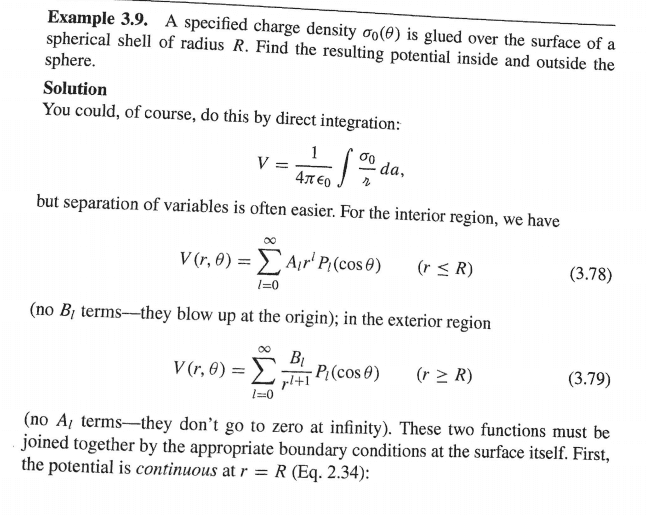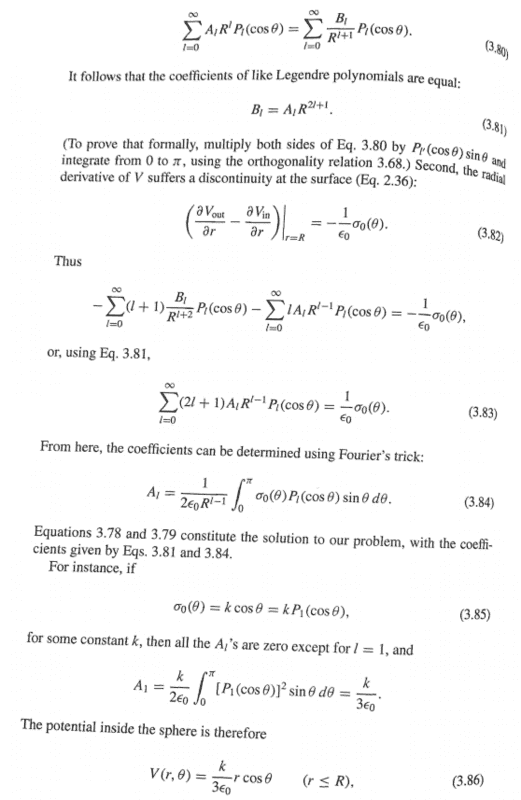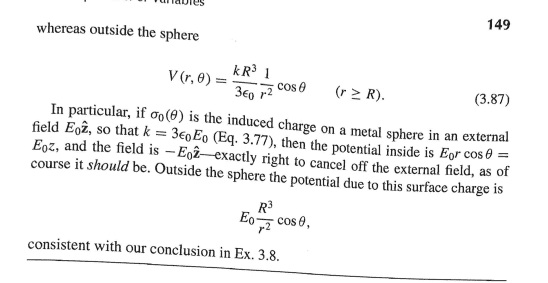I guess the reasoning isn't 100% there in my head and if i don't have an actual σ, how will i cancel out any legendre polynomials due to orthogonality?

Last edited by a moderator:

Homework Statement:: I need help on this question that i thought i understood but i really dont. A semi similar example in the book started to spark some brain juice but i ended up more confused than when i started.
Relevant Equations:: Legendre polynomials

View attachment 280846
So here was my first go around at it:
View attachment 280849
At first it made sense in my head but don't think my process is correct. Then i noticed the example in the book:
View attachment 280850
View attachment 280851
View attachment 280852
I guess the reasoning isn't 100% there in my head and if i don't have an actual σ, how will i cancel out any legendre polynomials due to orthogonality?
You cannot cancel any Legendre polynomials without knowing σ(θ). Take two simple cases that you can do in your head.
1. Uniform density over the sphere implies a 1/r potential and only P0 is non-zero.
2. σ(θ)=σ0cosθ implies a 1/r2 potential and only P1 is non-zero.

In short, if you don't know the surface charge distribution and you don't know the potential, you don't know nuttin'.

But you know the surface-charge distribution,
$$\sigma(\vartheta)=\sigma_0 \Theta(0 \leq \vartheta \leq \pi/2).$$
Now you can just use the solution for the coefficients posted in #1.

•vela and Orodruin